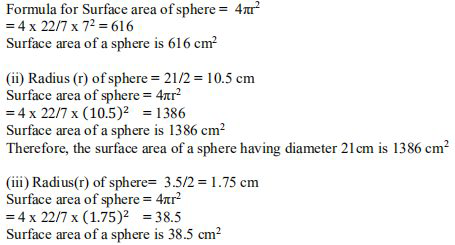# NCERT Solutions Class 9 Mathematics Solutions for Surface Areas and Volumes - Exercise 13.4 in Chapter 13 - Surface Areas and Volumes

Find the surface area of a sphere of diameter:

(i) 14 cm (ii) 21 cm (iii) 3.5 cm

(Assume π=22/7)

Radius of sphere , r = diameter/2 = 14/2 cm = 7 cmVideo transcript
"hello students welcome to lido q a video session i am seth your math tutor and question for today is find the surface area of the sphere of diameter first 14 centimeter second 21 centimeter and third 3.5 centimeter before beginning with the question here it is also given that assume y is equal to 22 by 7 so before beginning with the question let us say a general formula for the surface area of the sphere so general formula for the surface area of the sphere throughout the whole question we are going to use this formula that is 4 pi r square where r is the radius now let us take one by one each of this question the first question is 14 centimeter it is given that the diameter is 14 centimeter let us say d is equal to 14 centimeter and radius will be half of the diameter that is 14 upon 2 so radius is 7 centimeter here now applying the formula which is taken above that is surface area is equal to 4 pi r square 4 into 22 by 7 into 7 square so solving this you will get 616 unit is centimeter square let us take the second sub question in the second sub question diameter is 21 centimeter so let us take d is equal to 21 centimeter radius will be half of the diameter so radius will be 10.5 centimeter hence surface area is equal to 4 pi r square that is 4 into 22 by 7 into 10.5 whole square so this comes out to be after calculating you will get the value 1386 1386 square this is the surface area for the second question and the third one is 3.5 centimeter this is the diameter given so d is equal to 3.5 centimeter radius will be the half of the diameter 3.5 upon 2 you can calculate this and you will get 1.75 centimeter to be radius now once you have the radius you can apply the formula for the same surface area of the sphere that is taken above as a general one that is 4 pi into r square 4 into 22 by 7 into 1.75 whole square this will give you 38.5 centimeter square this is our final answer here so in all the three cases we have found surface area by applying the general formula for the surface area of the sphere that is 4 pi r square so if you have any query you can drop it in our comment section and subscribe to lido for more such q a thank you for watching "
Related Questions

Lido

Courses

Teachers

Book a Demo with us

Syllabus

Maths
CBSE
Maths
ICSE
Science
CBSE

Science
ICSE
English
CBSE
English
ICSE
Coding

Terms & Policies

Selina Question Bank

Maths
Physics
Biology

Allied Question Bank

Chemistry
Connect with us on social media!